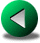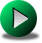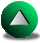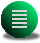Previous: Conclusions
Next: an A-grid energy conserving
Up: No Title

# Conclusions

In this thesis, we were interested in assessing the performance of different numerical methods for modelling the ocean in complex geometries. Complex geometries are represented by step-like walls in the most conventional numerical method used in oceanography, namely the finite different (FD) method. The presence of these steps may be detrimental to the representation of currents located along the boundaries, especially the western return currents if we consider the simple Munk gyre problem. From this perspective, finite element (FE) methods and spectral element (SE) methods with their accurate representation of the coastlines may provide more accurate solutions of the ocean circulation.

In Chapter 3, we compare these different numerical methods for a few test problems. In a rectangular geometry, the FD method is always more accurate at a given cost than FE methods using linear basis functions. However, for a simple analytical linear solution in a circular domain, we showed that conventional FD methods tend to have truncation orders between unity and two, instead of two. In that case FE methods provide more accurate solutions at the same cost than do FD methods. For nonlinear solutions and in a rectangular domain, all tested FE models showed a bias which tends to be robust with increasing resolution. In most finite element models, the problem is linked to numerical dissipative effects that were too small to be detected in the linear test-cases but that were large in the nonlinear test-case. These dissipative effects are related to the stability properties of each of the schemes and how each of them finds its way around the stability condition. Only one tested FE model satisfies to the so-called Ladyzhenskaya-Babuska-Brezzi (LBB) condition for FE models. This model showed also some signs of over-dissipation but in that case, the problem was more related to the use of a semi-Lagrangian treatment of the time discretization of the equations. Unfortunately, we have not made use of a FE model satisfying to the LBB condition with an Eulerian treatment of the equations. However, we can speculate based on the results of Chapter 3 that such a model would not be as cost-effective as the tested SE model. Nonetheless, the use of a LBB-complying FE model may prove to be more appropriate than FD models when spatially variable mesh capabilities are required, such as for resolving straits and inlets.

The tested element model shows high order truncation errors for linear and nonlinear test-cases in rectangular and circular domains. In the latter case, we reached a limitation due to our use of piecewise parabolas for the description of curved geometries. To test cost-effectiveness, we compared the SE and FD models for the nonlinear Munk problem in a rectangular domain. This test reveals that the accuracy of the SE method has to be about 1% of the true solution to be more effective than the second order C-grid FD model. However, for nonlinear problems the SE method presents a decisive advantage that its accuracy remains more or less identical in rectangular and generally curved domains whereas that of the finite difference methods degrades.

Finally, we tested with success an adaptive mesh strategy in a time-stepping mode. We designed an automated procedure that estimates the local error and refines the mesh accordingly in regions of largest errors as the simulation runs. This was tested for the Munk gyre problem. We noted that, when the required accuracy was high enough, most of the refinement goes into resolving the initial Kelvin waves which is excited by the onset of the wind and propagate along the boundary. After this initial transient process comes to rest, the mesh is automatically derefined along the boundary. In terms of cost, the adaptive procedure proves to be slightly less efficient than to run the model on a fixed mesh in time. However, this procedure might be useful in contexts for which the solution is not known a priori. In such cases, the location of sharp fronts is not known and may require a tedious manual remeshing in order to resolve these features. Sometimes, this process has to be done iteratively a large number of times and, in such a case, an adaptive strategy will prove far superior.

In Chapter 4, we focused on the influence of step-like walls in the finite difference methods, extending the study of Adcroft and Marshall. We used vorticity budgets as a diagnostic tool in order to assess the accuracy of the numerical solutions. We showed that the accuracy of FD methods degrades in presence of steps along the boundaries and that the truncation order is lowered. Depending on the specific numerics, we estimated that the truncation error varies between the zeroth and second order. In general, we found that vorticity budgets are not very accurate due to the presence of extra terms, such as the advection of vorticity, which do not appear in the analytical budget. Surprisingly, we noted that a quasi-geostrophic model does not lead to significantly more accurate vorticity budgets than those given by shallow water models, even though the former type of models explicitly solves for the vorticity equation. We also used a vorticity budget analysis on a shallow water B-grid model with free-slip boundary conditions which proved not to converge to a steady state with time, whereas the equivalent C-grid model does. In fact, this particular B-grid implementation proved to be inadequate.

In Chapter 5, we explored the theoretical possibility that free-slip circulations can develop their own eddies if the coastline is curved enough. This chapter can be considered as an application of the spectral element method and a contribution to the understanding of the ocean circulation from a theoretical point of view. Since it is not clear what type of boundary conditions is the most realistic to use at typical or even high resolution in ocean modelling, there is no obvious reason to discard the free-slip boundary condition6.1. So far, time dependent simulations of the nonlinear Munk problem in rectangular domains under free-slip boundary conditions show that the solution is very steady once it reaches its equilibrium. That is, no eddies develop. Moreover, under the same boundary condition, the solution becomes completely unrealistic passed a certain Reynolds number and still remains steady. Hence, the necessary eddies that transport the excess of vorticity to the walls are absent in these simulations. Therefore, these simulations in rectangular domains make a good case against the use of the free-slip boundary condition. This was certainly a strong incentive to use instead the no-slip boundary condition. However, the real oceans present irregular coastlines which may be the key-factor absent from these earlier experiments. We therefore investigated the influence of having curvy coastlines in the otherwise usual Munk problem for varying Reynolds number. The only model available to us that could perform such a task with a high degree of accuracy was the SE model. The finite difference models are too sensitive to the presence of steps along the coastline and the tested FE models are too dissipative for the Reynolds numbers we are interested in.

From scaling arguments and assuming a steady state with no transient eddies, we were able to derive that the bumps along the coastline cause the circulation to slow down compared to the no-bump case. This was due to the production of positive relative vorticity at the walls close to the tip of the bumps for a mid-latitude gyre in the northern hemisphere6.2. Furthermore, as the Reynolds number increases, we predicted that the total kinetic energy should increase at much slower rate than that of the no-bump case. If these free-slip circulations were able to produce their own eddies, it would be possible that even lower total kinetic energy values could be reached. Therefore, the presence of bumps along the coastline might be sufficient so that the main circulation escapes the anti-intuitive fate of not converging to some statistical steady state as the eddy viscosity is decreased. This fate is known as the inertial runaway and represents our inability to explain how the nonlinear processes of simple flows are sufficient to balance a decrease of poorly known diffusive parameters, such as the eddy viscosity.

Unfortunately, only the first prediction was verified; that the rate of increase of the total kinetic energy with increasing Reynolds number was decreased, but not reversed, contrary to our second hypothesis. We also noted a dependence on the local curvature of the coastline. The higher the curvature, the lower the total kinetic energy. Except for the largest curvature, the solutions jump to an unrealistic state passed a critical Reynolds number. For the largest curvature and the largest Reynolds number, we observe some eddy activity but not enough to slow down the total kinetic energy increase compared to our scaling arguments. In fact, most of the vorticity balance seems to be achieved by the main circulation. Indeed, the vorticity is large and positive along a significant portion of the bumps which leads to a large flux of vorticity at the walls. Moreover, we observed that the vorticity tends to follow a rather singular behavior along the bumps even though the bumps are smoothly curved. This was verified by using an adaptive mesh algorithm which increases the resolution of the model where the errors are the largest. More eddies could have been generated by larger curvature. However, we feared that we reached the validity limit, in terms of length scales of the observed processes, of the simple equations we were using, namely the shallow water equations. Baroclinic models may be required to represent the small scales features occurring along the western boundary.

One other important limitation of this study that we need to mention is related to the fractal nature'' of the coastline. From that perspective, it is quite unreasonable to define one'' curvature of the coastline, as this one is modified with increasing sampling of the coastline. Rather, we limit ourselves to the study of the influence of curved coastlines whose radius of curvature falls in the range of scales of interest (from the radius of deformation and the boundary layer widths to the basin scale). A more realistic approach would be to use a spectrum of wavelengths and amplitudes consistent with realistic coastlines. The overall result might not be very different from those presented in this thesis, though.

The SE model showed great advantages as a tool in order to address theoretical issues such as the inertial runaway problem. As it captures some features of the coastline, such as the curvature, we could address the issue of free-slip flows in presence of curved coastlines. However, its general variable resolution coupled to an adaptive mesh refinement enables this model to address the runaway problem and other theoretical aspects linked to nonlinear flows in presence of irregular coastlines for any kind of boundary condition. Nonetheless, the model still has to prove its effectiveness in the more general baroclinic framework. The vertical representation is certainly a very complex issue, and different strategies are possible. Schematically, the vertical representation can be z- or- or isopycnal levelled. Of the three, theseems to be the most natural to the SE method because it allows for a polynomial description in the vertical as well. However, it does not offer a good control on the particular depth range to resolve. It therefore may have difficulties in resolving sharp temperature or salinity gradients and may lead to Gibbs oscillations. The same problem exists in low-order numerical methods, such as finite difference models. However, it simply leads to accuracy problems rather than stability problems.

This study was obviously biased in focusing on one particular boundary condition, the free-slip boundary condition. Under this boundary condition, it is known that the FD methods do poorly in presence of steps. It may therefore seem obvious that SE methods do better. One may ask about the other well known boundary condition, the no-slip condition. For the no-slip boundary condition, we may assume that the FD methods in irregular domains do as well as they do for the nonlinear Munk problem in a rectangular domain (Section 3.4). In the latter case, we showed that the SE model does better only for high enough resolutions at which the error is below 1%. At this range of resolution, the error is low enough that FD methods are still competitive. Unless one is interested in representing accurately the fast transients of the ocean such as Kelvin waves, for which the FD methods do poorly independently of the boundary condition, the FD methods have still a long future in front of them. This last statement is also biased by the assumption governing the primitive equations. If, for instance, faster and bigger computers allow for very high resolution non-hydrostatic simulations (which require the inversion of a 3D matrix problem), then the FE methods might be attractive again, since their main overhead, consisting of the inversion of a matrix problem even when the equations are solved explicitly in time, is no more.

The last point we would like to mention is related to the effect of real'' steps present along the coastline as opposed to fake steps'' that FD discretization tend to generate. As they are singular features, no numerical method is able to model them, although some analytical approaches were proposed (Cherniawsky and Leblond, 1986). Nevertheless, real steps can be approached as the limit of increasing to infinity the curvature of the bumps. From that point of view, we can derive some qualitative conclusions based on the results obtained in Chapter 5 with the SE model. It seems that steps always have a dissipative effect and that all the fields will be singular close to the step. Therefore, the corrected version of Adcroft and Marshall (the B combination of Chapter 4) is biased because it under-represents the effect of steps by assuming that they are non-existent to the point that circulations in rotated basins look similar to circulation in non-rotated basins. Hence, their method is very successful in rotated rectangular domains but fails in more generally irregular domains. The correct solution in presence of irregular domains depends on the irregularity of the domain. It lies between the corrected version of Adcroft and Marshall and the traditional implementation of the C-grid model which is more dissipative. Ultimately, the true solution reflects the fractal nature of the coastline.Previous: Conclusions
Next: an A-grid energy conserving
Up: No Title

Frederic Dupont
2001-09-11